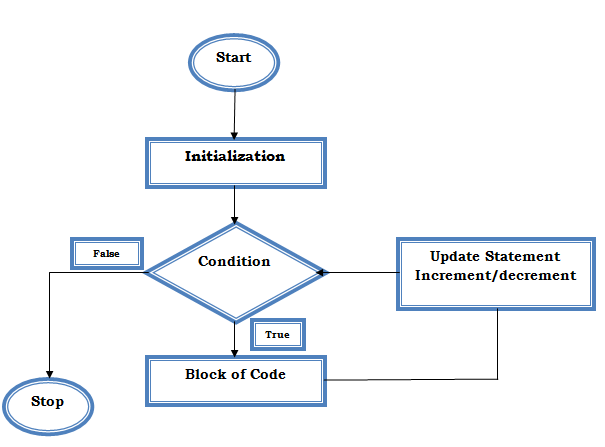## Description: For loop C

For loop execute a block of code number of times (repeatedly). For loop is a looping statement in the C programming language, which allows the code to be executed repeatedly until the condition becomes false. It has three parts:

• Initialization
• Condition
• Update statement (Increment or Decrement)

Syntax

for (initialization; condition; update statement)
{

//Block of Code

}

### FLOW DIAGRAM OF FOR LOOP CFlow Diagram of for loop C

Initialization: This step is executed first and it is executed only once when we are entering the loop for the first time. This step allows you to declare and initialize loop control variables.

Condition: Next step after initialization is to check the condition if the condition is true, it must return a boolean value. It is an entry controlled loop so the condition will check before entering into the block of code.

Update statement: After execution of the loop body, increment or decrement step is executed. This statement updates any loop variable.

### Control flow of for loop C

• First, initialize the variable 'i=1', It executes only once when we are entering into the loop.
• In the second step check condition i.e. 'i<=5'.
• In the third step, control goes inside the loop body and execute (print Hello five times).
• Next step is to increase the value of the variable i.e. (i=i+1 i.e i=2).
• Repeat above four steps until the condition becomes false.

### Common pitfalls in using for loop C

• A valid expression should be written in for loop statement otherwise it can lead to an infinite loop. The statement,

for ( ; ; )

// C program to illustrate infinite loop

#include <stdio.h>
int main ( )
{
for ( ; ; )
{
printf ("Hello");
}
return 0;
}

Output: This code prints the statement "Hello" repeatedly (infinite times).

• Many variables can be initialized in the initialization block of for loop, whether you use it in the loop or not.

//Program to illustrate initializing many variables in the initialization block.

#include <stdio.h>
int main ( )
{
int x = 2;
for (int y = 0, z = 4; x < 10 && y < 10; x++, y++)
{
printf (" x=%d y=%d \n", x, y);
}
return 0;
}

Output

x=2 y=0
x=3 y=1
x=4 y=2
x=5 y=3
x=6 y=4
x=7 y=5
x=8 y=6
x=9 y=7

Two variables are initialized and declared in initialization block, in which one variable i.e. 'z' is not used.

• The variables that are declared in the initialization block can be accessed only within the loop.

//Program to illustrate scope of initializing variables within the loop

#include <stdio.h>
int main ( )
{

for (int x = 3; x < 7; x++)
{
printf (" x=%d\n", x);
}

printf "x=%d\n", x );
return 0;
}

Output

error: 'x' undeclared

In the above example, variable x is not accessible outside the loop. The statement which is commented gives a compile error.

for loop CReviewed by Glory on 09:34 Rating: 5